# 22Strategy

### Summaries

• "Define a family of algorithms, encapsulate each one, and make them interchangeable. Strategy lets the algorithm vary independently from the clients that use it." [Gamma, p315]
• Capture the abstraction in an interface, bury implementation details in derived classes.

• [Before and After] Sort algorithms (C++)
• [Non-Software Example] The algorithm/abstraction of "getting to the airport"
• [Example 1] Format date algorithms (Java)
• [Example 2] Java's Comparator interface (Java)
• [Example 3] C++ function objects (C++)

### Motivation

One of the dominant strategies of object-oriented design is the "open-closed principle".

Software entities (classes, modules, functions, etc.) should be open for extension, but closed for modification. [Meyer, p23]
Figure 21-1 demonstrates how this is routinely achieved - encapsulate interface details in a base class, and bury implementation details in derived classes. Clients can then couple themselves to an interface, and not have to experience the upheaval associated with change: no impact when the number of derived classes changes, and no impact when the implementation of a derived class changes.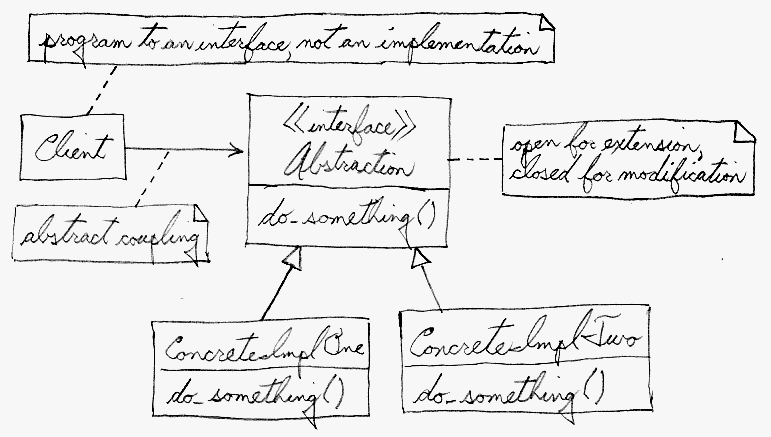Figure 21-1. An "open-closed principle" approach

A generic value of the software community for years has been, "maximize cohesion and minimize coupling". The object-oriented design approach shown in Figure 21-1 is all about minimizing coupling. Since the client is coupled only to an abstraction (i.e. a useful fiction), and not a particular realization of that abstraction, the client could be said to be practicing "abstract coupling" . an object-oriented variant of the more generic exhortation "minimize coupling".

A more popular characterization of this "abstract coupling" principle is ...

Program to an interface, not an implementation.
Clients should prefer the "additional level of indirection" that an interface (or an abstract base class) affords. The interface captures the abstraction (i.e. the "useful fiction") the client wants to exercise, and the implementations of that interface are effectively hidden.

The Strategy design pattern discusses and documents the leverage represented in Figure 21-1. Other terms that are part and parcel of this same concept are: polymorphism, and dynamic binding.

### Structure Summary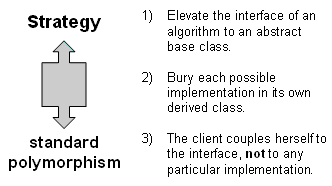Structure category:
inheritance hierarchy
Similar patterns:   Factory Method   Visitor

### Before and After

The class Stat below takes an array of integers and can return the min, max, and median values present. Its implementation is inextricably coupled to an embedded bubble sort algorithm.
```class Stat {
public:
void readVector( int v[], int n ) {
sort( v, n );
m_min = v;
m_max = v[n-1];
m_median = v[n/2];
}
int getMin()    { return m_min; }
int getMax()    { return m_max; }
int getMedian() { return m_median; }
private:
int m_min, m_max, m_median;
void sort( int v[], int n ) {     /* Bubble sort */
for (int i=n-1; i > 0; --i)
for (int j=0; j < i; ++j)
if (v[j] > v[j+1]) {
int t = v[j];
v[j] = v[j+1];
v[j+1] = t;
}
cout << "Bubble: ";
for (int k=0; k < n; ++k)
cout << v[k] << ' ';
cout << '\n';
}
};

int main( void ) {
const int NUM = 9;
int       array[NUM];
srand( time(0) );
cout << "Vector: ";
for (int i=0; i < NUM; ++i) {
array[i] = rand() % 9 + 1;
cout << array[i] << ' ';
}
cout << '\n';

Stat  obj;
cout << "min is " << obj.getMin() << ", max is " << obj.getMax()
<< ", median is " << obj.getMedian() << '\n';
}

// Vector: 6 9 9 8 6 5 7 9 2
// Bubble: 2 5 6 6 7 8 9 9 9
// min is 2, max is 9, median is 7
```
The choice of sort algorithm is not intrinsic to the abstraction of Stat; all that Stat wants is some component capable of sorting an array of integers. If the choice of sort algorithm were configurable, then users of Stat could decide for themselves what kind of "time versus space" trade-offs they require.

Let's abstract the notion of sort and represent that abstraction with an interface. Stat will now "program to an interface" by holding and referencing a pointer to that interface. How the pointer is initialized will be addressed later.

```class SortImpl {
public:
virtual void sort( int[], int ) = 0;
};

class Stat {
public:
void readVector( int v[], int n ) {
m_impl->sort( v, n );
m_min = v;
m_max = v[n-1];
m_median = v[n/2];
}
...
private:
SortImpl*  m_impl;
};
```
Our bubble sort algorithm can then declare itself to be a concrete implementation of the abstract interface.
```class SortBubble : public SortImpl {
public:
void sort( int v[], int n ) {
for (int i=n-1; i > 0; --i)
for (int j=0; j < i; ++j)
if (v[j] > v[j+1]) {
int t = v[j];
v[j] = v[j+1];
v[j+1] = t;
}
cout << "Bubble: ";
for (int k=0; k < n; k++)
cout << v[k] << ' ';
cout << '\n';
}
};
```
With the client coupled only to the SortImpl interface, our design is now "open for extension". Let's demonstrate that new-found leverage by adding a shell sort algorithm.
```class SortShell : public SortImpl {
public:
void sort( int v[], int n ) {
for (int g = n/2; g > 0; g /= 2)
for (int i = g; i < n; ++i)
for (int j = i-g; j >= 0; j -= g)
if (v[j] > v[j+g]) {
int temp = v[j];
v[j] = v[j+g];
v[j+g] = temp;
}
cout << "Shell:  ";
for (int k=0; k < n; k++)
cout << v[k] << ' ';
cout << '\n';
}
};
```
Rather than hard-wire the choice of sort algorithm in the Stat class, we can choose a default implementation in the constructor, and allow the client of Stat to change it at will.
```class Stat {
public:
Stat() {
m_impl = new SortBubble;
}
delete m_impl;
m_impl = new SortShell;
}
delete m_impl;
m_impl = new SortBubble;
}
...
private:
SortImpl*  m_impl;
};
```
`main()` is now changed to exercise the new `upGrade()` and `downGrade()` methods. The output appears at the end.
```int main( void ) {
const int NUM = 9;
int       array1[NUM], array2[NUM];
srand( time(0) );
cout << "Vector: ";

for (int i=0; i < NUM; ++i) {
array1[i] = array2[i] = rand() % 9 + 1;
cout << array1[i] << ' '; }
cout << '\n';

Stat  obj;
cout << "min is " << obj.getMin() << ", max is " << obj.getMax()
<< ", median is " << obj.getMedian() << '\n';
cout << "min is " << obj.getMin() << ", max is " << obj.getMax()
<< ", median is " << obj.getMedian() << '\n';
}

// Vector: 7 4 2 6 5 7 7 5 1
// Shell:  1 2 4 5 5 6 7 7 7
// min is 1, max is 7, median is 5
// Bubble: 1 2 4 5 5 6 7 7 7
// min is 1, max is 7, median is 5
```
When we defined our interface in a base class, buried implementation in derived classes, and coupled the client to the abstraction represented by the interface, we exercised the object-oriented feature called polymorphism. My favorite definition of polymorphism is
one interface, many shapes (or implementations)
It is also referred to as run-time (or dynamic) polymorphism, because the code generated by the compiler has sufficient indirection built-in so that the choice of sort algorithm can be decided at run-time (instead of having to be declared at compile-time).

An alternate implementation approach for the Strategy pattern uses C++ templates. Templates have been described as compile-time (or static) polymorphism. Consider the following code example. The only expectations the OutsideClass places on the delegate object are: must have a do_one() method, and must have a do_two() method.

```class InsideClass {
public:
void do_one() { cout << "InsideClass::do_one\n"; }
void do_two() { cout << "InsideClass::do_two\n"; }
};

class OutsideClass {
public:
void do_it() {
delegate.do_one();
delegate.do_two();
}
private:
InsideClass delegate;
};

int main( void ) {
OutsideClass object;
object.do_it();
}

// InsideClass::do_one
// InsideClass::do_two
```
C++ templates allow "data types" referenced by a class "to be supplied" by the client of that class. Here is the syntax for a class template.
```template<typename TBS>
class OutsideClass {
public:
void do_it() {
delegate.do_one();
delegate.do_two();
}
private:
TBS delegate;
};
```
A C++ compiler that supports templates is capable of parsing this source code, identifying the do_one() and do_two() requirements, and allowing any class that satisfies these requirements to be specified by the client. A new class and the client code appear below.
```class AlternateClass {
public:
void do_one() { cout << "AlternateClass::do_one\n"; }
void do_two() { cout << "AlternateClass::do_two\n"; }
void do_thr() { cout << "AlternateClass::do_thr\n"; }
};

...

int main( void ) {
OutsideClass object1;
object1.do_it();
OutsideClass object2;
object2.do_it();
}

// InsideClass::do_one
// InsideClass::do_two
// AlternateClass::do_one
// AlternateClass::do_two
```
C++ templates are useful if the type of referenced objects are known at compile-time, and those objects do not need to be swapped with other objects from the same inheritance hierarchy at any point during run-time. Let's try a template out on our previous sort example.
```template<typename STRATEGY>
class Stat {
public:
void readVector( int v[], int n ) {
m_impl.sort( v, n );
m_min = v;
m_max = v[n-1];
m_median = v[n/2];
}
...
private:
STRATEGY  m_impl;
};
```
When a template is used, there is no need to create a "lowest common denominator base class" that makes all derived classes interchangeable. In this case, any class that provides a method with the signature void sort(int[],int) can be supplied to the Stat class.
```class SortBubble {
void sort( int v[], int n ) {
...

class SortShell {
void sort( int v[], int n ) {
...

int main( void ) {
const int NUM = 9;
int       array[NUM];
srand( time(0) );
cout << "Vector: ";
for (int i=0; i < NUM; ++i) {
array[i] = rand() % 9 + 1;
cout << array[i] << ' ';
}
cout << '\n';
Stat<SortBubble>  one;
cout << "min is " << one.getMin() << ", max is " << one.getMax()
<< ", median is " << one.getMedian() << '\n';

Stat<SortShell>  two;
cout << "min is " << two.getMin() << ", max is " << two.getMax()
<< ", median is " << two.getMedian() << '\n';
}

// Vector: 8 3 1 9 7 2 2 9 7
// Bubble: 1 2 2 3 7 7 8 9 9
// min is 1, max is 9, median is 7
// Shell:  1 2 2 3 7 7 8 9 9
// min is 1, max is 9, median is 7
```
The Strategy design pattern is about making alternate implementations of an algorithm interchangeable, and coupling the client of that algorithm to an interface only. In C++ the designer has two approaches to consider: dynamic polymorphism using a virtual function in an inheritance hierarchy, or static polymorphism using a class template.

### Non-Software Example

"A Strategy defines a set of algorithms that can be used interchangeably. Modes of transportation to an airport is an example of a Strategy. Several options exist such as driving one's own car, taking a taxi, an airport shuttle, a city bus, or a limousine service. For some airports, subways and helicopters are also available as a mode of transportation to the airport. Any of these modes of transportation will get a traveler to the airport, and they can be used interchangeably. The traveler must chose the Strategy based on tradeoffs between cost, convenience, and time." [Duell, p54]Figure 21-2. Choice of "go to airport" algorithm

### StructureFigure 21-3. Decouple client from algorithm implementation

The Interface entity in Figure 21-3 could represent either an abstract base class, or the method signature expectations by the client. In the former case, the inheritance hierarchy represents dynamic polymorphism. In the latter case, the Interface entity represents template code in the client and the inheritance hierarchy represents static polymorphism.

### Behavior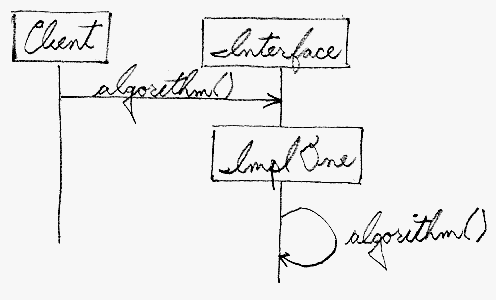Figure 21-4. Client talks to Interface, Implementation actually responds

Figure 21-4 is faux UML. The intent is to demonstrate that the client is coupled only to the Interface, but the actual recipient of any request is whichever implementation class has been instantiated.

### Summary

1. Identify an algorithm (i.e. a behavior) that the client would prefer to access through a "flex point".
2. Specify the signature for that algorithm in an interface.
3. Bury the alternative implementation details in derived classes.
4. Clients of the algorithm couple themselves to the interface.

### Example 1

Formatting dates is a fairly popular algorithm that has been encapsulated many times in the past. Let's use this domain as a vehicle for discussing the Strategy pattern. It would be nice if . we had a polymorphic capability of producing the following kinds of date formats.
```October 2, 2005
2 Oct 2005
10/2/05
02/10/05
20051002
2005275
```
Consider a BillingSystem that needs to format dates - and - wants the format used to be configurable. The code below simulates the "client" of a DateFormatStrategy abstraction.
```interface DateFormatStrategy {
String format_date( Date d );
}

class BillingSystem {
private DateFormatStrategy formatter = null;
public  void set_format( DateFormatStrategy dfs ) {
formatter = dfs;
}
public  void print_statement( Date now ) {
if (formatter == null)
System.out.println( now.toString() );
else
System.out.println( formatter.format_date( now ) );
}  }

public class StrategyDemo {
public static void main( String[] args ) {
BillingSystem the_client = new BillingSystem();
Date today = new Date();
the_client.print_statement( today );
the_client.set_format( new ShortUnitedKingdomStrategy() );
the_client.print_statement( today );
}  }

// Mon Oct 03 21:58:08 CDT 2005
// 03/10/05
```
Figure 21-5 captures the relationships and decoupling we intend to implement.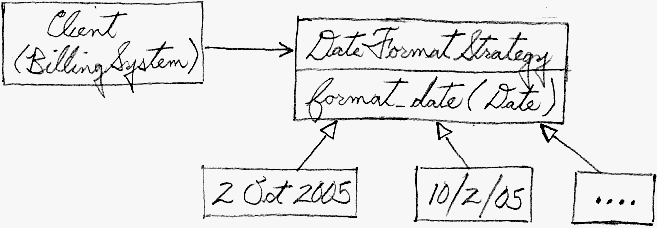Figure 21-5. Encapsulating the Date formatting algorithm

A possible suite of DateFormatStrategy implementations follows. Given the richness of the Java standard library, it could be argued that there is not sufficient justification to create a new Strategy inheritance hierarchy for so little leverage. The significance of this example though, is not the "letter of the implementation", it is the "spirit of the pattern".

```class ShortUnitedStatesStrategy implements DateFormatStrategy {
private DateFormat df = DateFormat.getDateInstance( DateFormat.SHORT );
public String format_date( Date d ) {
return df.format( d );
}  }
class ShortUnitedKingdomStrategy implements DateFormatStrategy {
private DateFormat df = DateFormat.getDateInstance(
DateFormat.SHORT, Locale.UK );
public String format_date( Date d ) {
return df.format( d );
}  }
class SortableStrategy implements DateFormatStrategy {
private DateFormat df = new SimpleDateFormat( "yyyyMMdd" );
public String format_date( Date d ) {
return df.format( d );
}  }
class SubtractableStrategy implements DateFormatStrategy {
private DateFormat df = new SimpleDateFormat( "yyyyDDD" );
public String format_date( Date d ) {
return df.format( d );
}  }
```
These Strategy implementations can be tested polymorphically with the following client code.
```public class StrategyDemo {
public static void main( String[] args ) {
Date now = new Date();
DateFormatStrategy[] strats = { new ShortUnitedStatesStrategy(),
new ShortUnitedKingdomStrategy(),
new SortableStrategy(),
new SubtractableStrategy() };
for (int i=0; i < strats.length; ++i)
System.out.println( strats[i].format_date( now ) );

// Test the julian date formatter
DateFormatStrategy dfs = new SubtractableStrategy();
Calendar cal = new GregorianCalendar();
cal.set( Calendar.MONTH, 1 );
cal.set( Calendar.DAY_OF_MONTH, 1 );
int first = Integer.parseInt( dfs.format_date( cal.getTime() ) );
cal.set( Calendar.MONTH, 2 );
int second = Integer.parseInt( dfs.format_date( cal.getTime() ) );
System.out.println( "" + second + " - " + first + " = "
+ (second - first) );
}  }

// 10/2/05
// 02/10/05
// 20051002
// 2005275
// 2005060 - 2005032 = 28
```
Let's go back to the original BillingSystem implementation.
```class BillingSystem {
...
public  void print_statement( Date now ) {
if (formatter == null)
System.out.println( now.toString() );
else
System.out.println( formatter.format_date( now ) );
}  }
```
The if...else construct that tests whether the formatter object has been initialized is problematic. Right now that test only occurs in this one place. But - as the application evolves and grows, anybody that uses the formatter object will have to perform this same test. It would be nice if this brute-force, state-oriented, C-style of "test and branch" programming could be encapsulated within the DateFormatStrategy "extra level of indirection" that we already have in place.

There is a non-gang-of-four pattern that addresses this kind of opportunity - the Null Object Pattern.

A Null Object simplifies the client by relieving it of having to write special testing code to handle "lack of initialization" situations. It encapsulates the implementation decisions of how to do nothing and hides those details from the client. [Woolf, pxxx]
The idea is to "promote to full object status" whatever it means to be un-initialized. In this case, the now.toString() statement can become a class of its own, and the formatter attribute can be assigned a default value of new NullStrategy. Notice that the print_statement() method can now delegate to the formatter object without first checking to see if it has been initialized. Removing extraneous control logic is always a good thing.
```class NullStrategy implements DateFormatStrategy {
public String format_date( Date d ) {
return d.toString();
}  }
...
class BillingSystem {
private DateFormatStrategy formatter = new NullStrategy();
...
public void print_statement( Date now ) {
System.out.println( formatter.format_date( now ) );
}  }
```

### Example 2

Consider Java's TreeSet class. It guarantees that all objects added will be maintained in ascending order. In the example below, the TreeSet object uses the compareTo() method is the containee class Struct to keep the elements sorted.
```class Struct implements Comparable {
public String first;
public String second;
public String third;
public Struct( String f, String s, String t ) { first=f; second=s; third=t; }
public int compareTo( Object other ) {
return first.compareTo( ((Struct)other).first );
}  }

public class StrategyDemo {
public static void main( String[] args ) {
TreeSet<Struct> one = new TreeSet<Struct>();
one.add( new Struct( "aaa", "dbb", "vcc" ) );
one.add( new Struct( "zaa", "ebb", "rcc" ) );
one.add( new Struct( "maa", "bbb", "tcc" ) );
one.add( new Struct( "saa", "abb", "ucc" ) );
one.add( new Struct( "gaa", "cbb", "scc" ) );
for (Struct s : one)
System.out.print( s.first + " " );
System.out.println();
}  }

// aaa gaa maa saa zaa
```
The relationships are portrayed in Figure 21-6. Note that the compare functionality that makes the sorting possible is fixed in the containee class. The user of TreeSet is not able to configure any alternate ordering.Figure 21-6. Compare functionality for sort in containee class

It would be nice if the "compare" algorithm were decoupled from the Struct class, and implemented as a Strategy. That would allow the user of the TreeSet class to choose from a menu of Strategy objects and customize the ordering of contained objects. Each CompareXxx class keys off of a different attribute of the Struct class.

```TreeSet<Struct> one = new TreeSet<Struct>( new CompareFirst() );
one.add( new Struct( "aaa", "dbb", "vcc" ) );
one.add( new Struct( "zaa", "ebb", "rcc" ) );
one.add( new Struct( "maa", "bbb", "tcc" ) );
one.add( new Struct( "saa", "abb", "ucc" ) );
one.add( new Struct( "gaa", "cbb", "scc" ) );
// report the "first" members of the "one" set
TreeSet<Struct> two = new TreeSet<Struct>( new CompareSecond() );
// populate the "two" set and report the "second" members
TreeSet<Struct> thr = new TreeSet<Struct>( new CompareThird() );
// populate the "thr" set and report the "third" members

// aaa gaa maa saa zaa
// abb bbb cbb dbb ebb
// rcc scc tcc ucc vcc
```
We have effectively refactored the "compare" functionality to full object status. It has migrated from the Struct class to a Strategy inheritance hierarchy.
```class Struct {
String first;
String second;
String third;
Struct( String f, String s, String t ) { first=f; second=s; third=t; }
}

class CompareFirst implements Comparator {
public int compare( Struct left, Struct rite ) {
return left.first.compareTo( rite.first );
}  }
class CompareSecond implements Comparator {
public int compare( Struct left, Struct rite ) {
return left.second.compareTo( rite.second );
}  }
class CompareThird implements Comparator {
public int compare( Struct left, Struct rite ) {
return left.third.compareTo( rite.third );
}  }
```
Figure 21-7 demonstrates this new "degree of freedom". TreeSet still contains Struct objects. But it now delegates "compare" functionality to the Strategy object with which it is configured.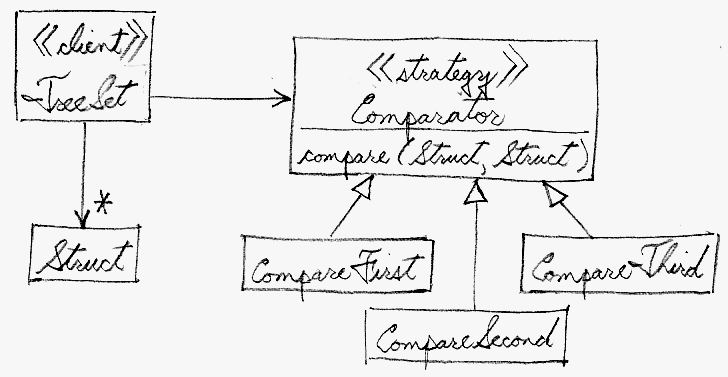Figure 21-7. "compare" is now a Strategy algoritihm

### Example 3

The C++ standard library sort() algorithm is a subtle analogy for the static polymorphism version of the Strategy design pattern. sort() is case sensitive by default. The results shown below demonstrate that a case insensitive sort would be nice.
```#include <algorithm>

int main( int argc, char* argv[] ) {
string array[] = { "The", "quick", "brown" , "Fox", "jumped",
"over", "the", "lazy", "Dog" };
const int SIZE = sizeof(array) / sizeof(string);
for (int i=0; i < SIZE; ++i)
cout << array[i] << ' ';
cout << '\n';

sort( array, array + SIZE );
for (int i=0; i < SIZE; ++i)
cout << array[i] << ' ';
cout << '\n';
}

// The quick brown Fox jumped over the lazy Dog
// Dog Fox The brown jumped lazy over quick the
```
Fortunately, the sort() algorithm will accept any "compare" algorithm that supports the correct "signature" - the function must return a bool, and accept two objects by const reference of the same type as the first two arguments to sort(). The implementation of no_case_sort_function() came from Stroustrup, p467.
```bool no_case_sort_function( const string& left, const string& rite ) {
string::const_iterator first  = left.begin();
string::const_iterator second = rite.begin();
while (first != left.end() && second != rite.end()
&& toupper(*first) == toupper(*second)) {
++first;
++second;
}
if (first == left.end())
return second != rite.end();
if (second == rite.end())
return false;
}

int main( int argc, char* argv[] ) {
...
// A function signature consists of: the return type, and the number and
// type of all arguments
bool (*ptr_to_func)(const string&, const string&) = &no_case_sort_function;
sort( array, array + SIZE, ptr_to_func );
for (int i=0; i < SIZE; ++i)
cout << array[i] << ' ';
cout << '\n';
}

// The quick brown Fox jumped over the lazy Dog
// brown Dog Fox jumped lazy over quick The the
```
A C++ alternative to the C function pointer is a "functor" or "function object". A functor is an instance of a class that defines the function call operator (aka, the application or parentheses operator). This feature effectively promotes to full object status the notion of a function invocation. The benefits of this "extra level of indirection" include: the traditional object-oriented leverage of co-locating state and behavior, and improved performance as a consequence of the inlining of code that will likely result.
```class no_case_sort_class {
public:
bool operator()( const string& left, const string& rite ) {
...   // the same compare logic from before
}
};

int main( int argc, char* argv[] ) {
...
// Create a temporary instance of the functor class, and pass it by
// value to the sort() algorithm
sort( array, array + SIZE, no_case_sort_class() );
for (int i=0; i < SIZE; ++i)
cout << array[i] << ' ';
cout << '\n';
}

// The quick brown Fox jumped over the lazy Dog
// brown Dog Fox jumped lazy over quick The the
```
Figure 21-8 presents an abstract characterization of these implementation alternatives. The letter of the UML law has been violated, but the spirit has been preserved. The sort() function has decoupled itself from the algorithm" of "compare". It is happy to delegate all compare functionality to any entity that can fulfill the TBD function signature. The user of sort() can specify at compile-time not only the compare algorithm, but also the choice of language feature.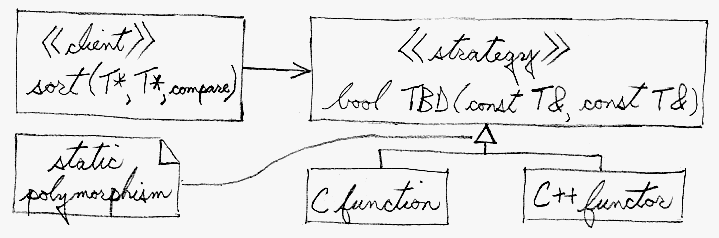Figure 21-8. sort() is coupled to a signature, not an implementation

### Heuristics

"Strategy is like Template Method except in its granularity." [CoplienMar96, p88]

"State is like Strategy except in its intent." [CoplienMar96, p88]

"Strategy lets you change the guts of an object. Decorator lets you change the skin." [Gamma, p184]

"State, Strategy, Bridge (and to some degree Adapter) have similar solution structures. They all share elements of the 'handle/body' idiom. They differ in intent - that is, they solve different problems." [Coplien, p58]

"Strategy has 2 different implementations, the first is similar to State. The difference is in binding times (Strategy is a bind-once pattern, whereas State is more dynamic)." [CoplienMar96, p88]

"Strategy objects often make good Flyweights." [Gamma, p323]

In C++, "policy classes" are "classes that encode an action that is largely orthogonal with respect to any other [flex point]." [Alexandrescu, p258] They ... capture and encapsulate reusable behavior that would otherwise proliferate. "Policies are reminiscent of the Strategy design pattern with the twist that policies are compile-time bound." [Alexandrescu, p8] The hardest part of creating policy-based class design is to correctly decompose the functionality of a class. The rule of thumb is ... anything that can be done in more than one way should be migrated from the class [and isolated as] a policy." [Alexandrescu, p19]